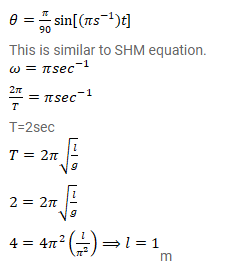# The angle made by the string of a simple pendulum with

Question:

The angle made by the string of a simple pendulum with the vertical depends on the time as $\theta=\frac{\pi}{90} \sin \left[\left(\pi s^{-1}\right) t\right]$.

Find the length of the pendulum if $g=\pi^{2} \mathrm{~m} / \mathrm{s}^{2}$.

Solution: